Study Guide

# Comparing Improper Integrals

## Comparing Improper Integrals

Usually it's more important to know whether an improper integral converges than it is to know what it converges to.

We can often figure out whether an improper integral converges or diverges by comparing it to another improper integral whose convergence / divergence we already know.

• ### The p-Test

We often use integrals of the functions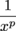, for various values of p, to help determine whether other integrals converge or diverge.

You already did the work to show this, so we'll just summarize the results. Assuming p is greater than 0 (because otherwise the exponents do weird things),

•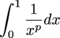converges if p < 1 and diverges otherwise.
•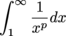converges if p > 1 and diverges otherwise.

This is often called the p-test for improper integrals.

• ### Finite and Infinite Areas

Besides the p-test, there are a few basic principles that go into the rest of this section.

If we have a convergent integral and we make its interval of integration smaller, the new integral will also converge.

Oddly enough, we can also make the interval of integration larger, as long as we don't include any points where the function is badly behaved. If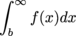converges and f isn't badly behaved on [a, b], then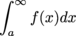also converges.

This is because we changed the original improper integral by sticking on a finite area, and a definite integral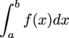that isn't improper is always finite.

On the other hand, if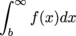diverges then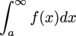will diverge also.

The moral of the story is that when we're looking at integrals of the form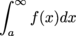,

it doesn't really matter what the lower limit of integration is so long as f (x) is well behaved on the interval of integration.

The integral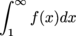will converge if and only if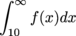converges, if and only if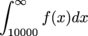converges.

### Sample Problem

We know that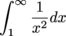converges. This means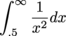also converges, because we've only added on a finite amount of area.

### Sample Problem

We know that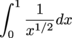converges. This means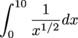also converges. The integral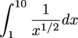is finite, so we've only increased the area by a finite amount.

If

0 ≤  f (x) < g(x)

for all x in [a, b] at which both functions are defined, and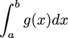converges, then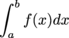must converge as well. The area under g on [a, b] includes the area under f on [a, b], so if the area under g is finite the area under f must be finite as well. Similarly, if 0 ≤  f (x) < g(x) for all x in [a, b] at which both functions are defined, anddiverges, then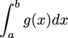must diverge as well. The area under g on [a, b] includes the area under f on [a, b], so if the area under f is infinite the area under g must be infinite as well.

If 0 ≤  f (x) < g(x) for all x in [a, b] at which both functions are defined, and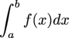converges, that doesn't help us figure out what the corresponding integral of g does. It doesn't help us to know that the area under g is larger than a finite area. Similarly, knowingdiverges doesn't help us figure out what the corresponding integral of f does. It doesn't help us to know that the area under f is smaller than an infinite area.

This property also extends to improper integrals with infinite limits.

If 0 ≤  f (x) < g(x) for all x in [a, ∞), then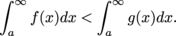If the integral of g is finite, so is the integral of f. If the integral of f is infinite, so is the integral of g.

• ### Comparison with Formulas

We can figure out whether integrals converge or diverge by comparing them with other integrals whose convergence or divergence we already know. When we're looking at formulas and not at graphs, we have to figure out from scratch what to compare an integral to.

• If we want to show that an integral converges, we have to find a larger function whose integral on the same interval converges.
• If we want to show that an integral diverges, we have to find a smaller function whose integral on the same interval diverges.